# C/C++ Program to the Count Inversions in an array using Merge Sort?

The Count of inversions that take place to Sort the given array is known as inversion count. the inversion problem is a classical problem that can be solved using the merge sort Algorithm. in this problem v we will count all elements more than it to its left and add the count to output. ThisLogic is done inside merge function of merge sort.

For understanding the topic better let us take an example. Let us consider two sub-arrays involved in merge process -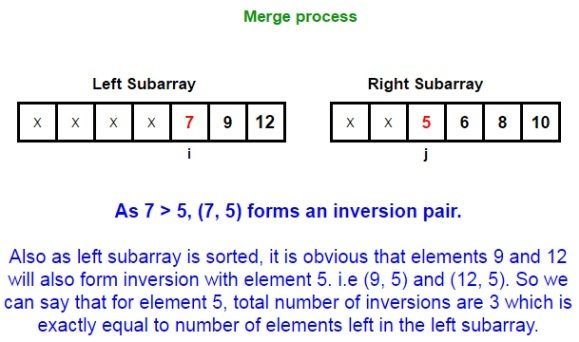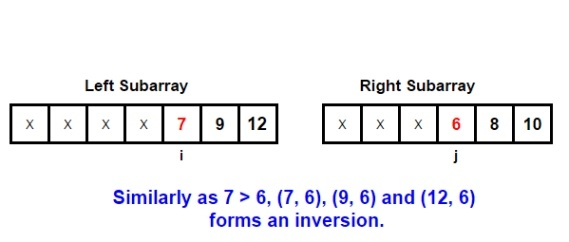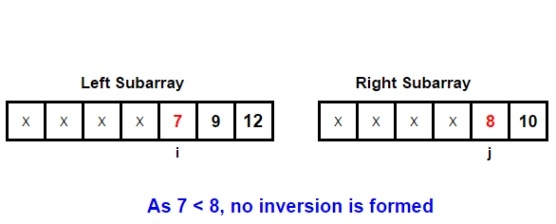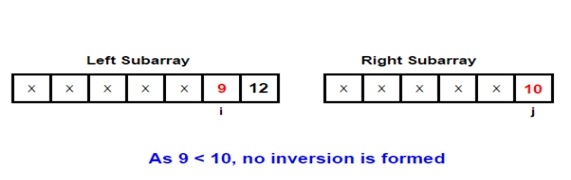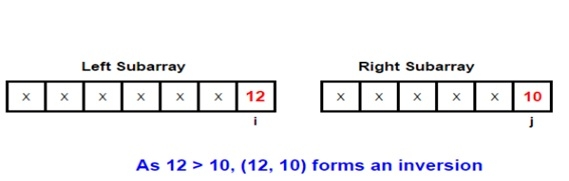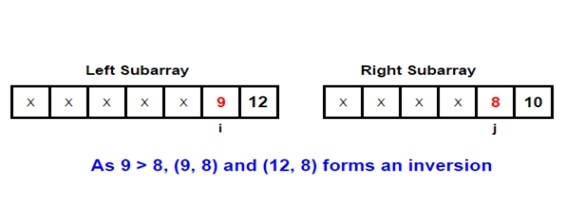Input: arr[] = { 1, 9, 6, 4, 5}
Output: Inversion count is 5

## Explanation

Inversion count of an array

Given an array, find the number of inversions of it. If (i < j) and (A[i] > A[j]) then the pair (i, j) is called an inversion of an array A. We need to count all such pairs in the arr

For example,

There are 5 inversions in the array

(9,6), (9,4), (9,5), (6,4), (6,5)

1. Compare the values of the element with each other.

2. Increment the counter if the value at lower index is higher.

3. Display the result.

## Example

#include <stdio.h>
int Merge(int arr[], int aux[], int low, int mid, int high) {
int k = low, i = low, j = mid + 1;
int inversionCount = 0;
while (i <= mid && j <= high) {
if (arr[i] <= arr[j]) {
aux[k++] = arr[i++];
} else {
aux[k++] = arr[j++];
inversionCount += (mid - i + 1); // NOTE
}
}
while (i <= mid)
aux[k++] = arr[i++];
for (int i = low; i <= high; i++)
arr[i] = aux[i];
return inversionCount;
}
int MergeSort(int arr[], int aux[], int low, int high) {
if (high == low) // if run size == 1
return 0;
int mid = (low + ((high - low) >> 1));
int inversionCount = 0;
inversionCount += MergeSort(arr, aux, low, mid);
inversionCount += MergeSort(arr, aux, mid + 1, high);
inversionCount += Merge(arr, aux, low, mid, high);
return inversionCount;
}
int main() {
int arr[] = { 1, 9, 6, 4, 5 };
int N = 5;
int aux[N];
for (int i = 0; i < N; i++)
aux[i] = arr[i];
printf("Inversion count is %d", MergeSort(arr, aux, 0, N - 1));
return 0;
}